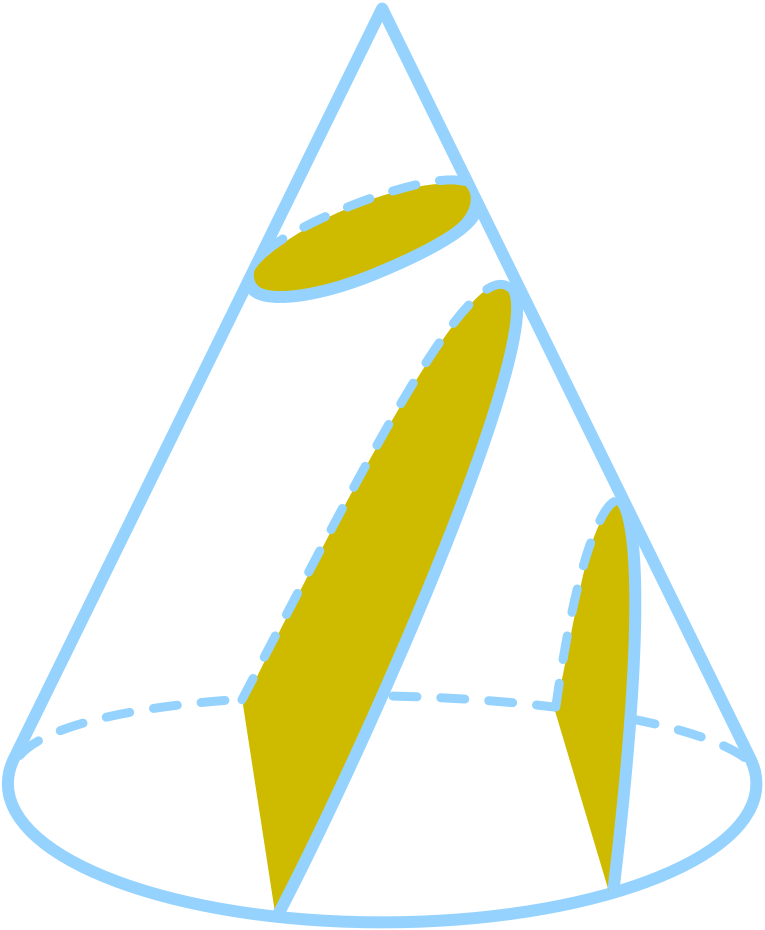# Orbits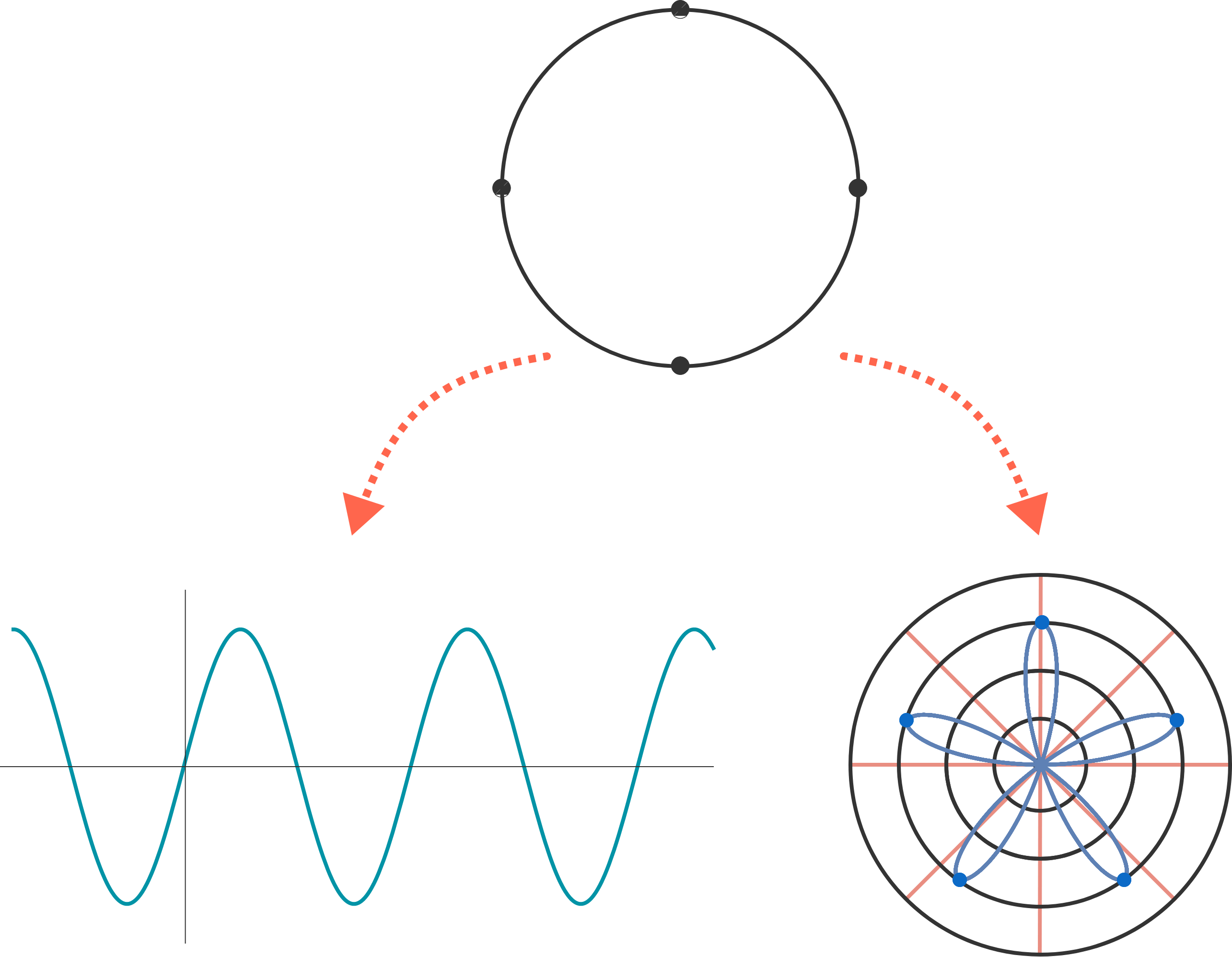The previous two quizzes derived the idea of sine waves and polar coordinates out of circles. Let's now change the shape of circles themselves into ellipses.

A circle is the set of points equidistant around a center. Rather than having a single center, an ellipse has two points called foci. The ellipse is the set of points such that, given any point $$P$$ on the ellipse, $$($$the distance from $$P$$ to one focus$$)$$ + $$($$the distance from $$P$$ to the other focus$$)$$ is the same.

In the picture below, the sum of the distances marked by matching colors is constant.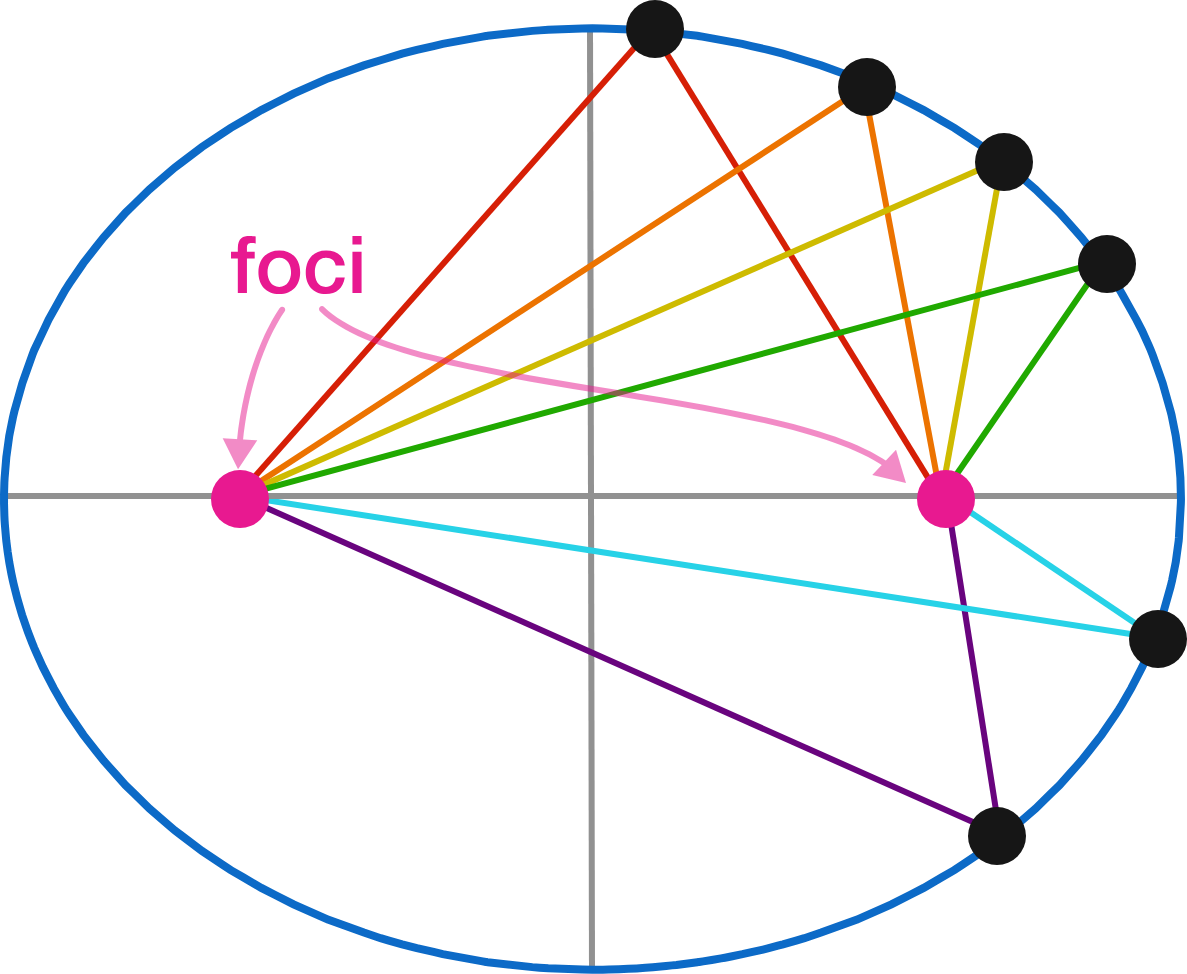## Orbits

### Introduction

# Orbits

Let's dig further, and work out what the equation for an ellipse (and as we'll get to, a planetary orbit) looks like. With circles, we've seen the equation $$x^2 + y^2 = r^2 ;$$ let's go back to that point and start again with just the unit circle $$x^2 + y^2 = 1 .$$ Then, put in a term $$Q,$$ as shown in the visualization below.

$(Qx)^2 + y^2 = 1$ The original unit circle measures 2 units across. If we want an ellipse that measures 8 units across, what should $$Q$$ be?

## Orbits

### Introduction

# Orbits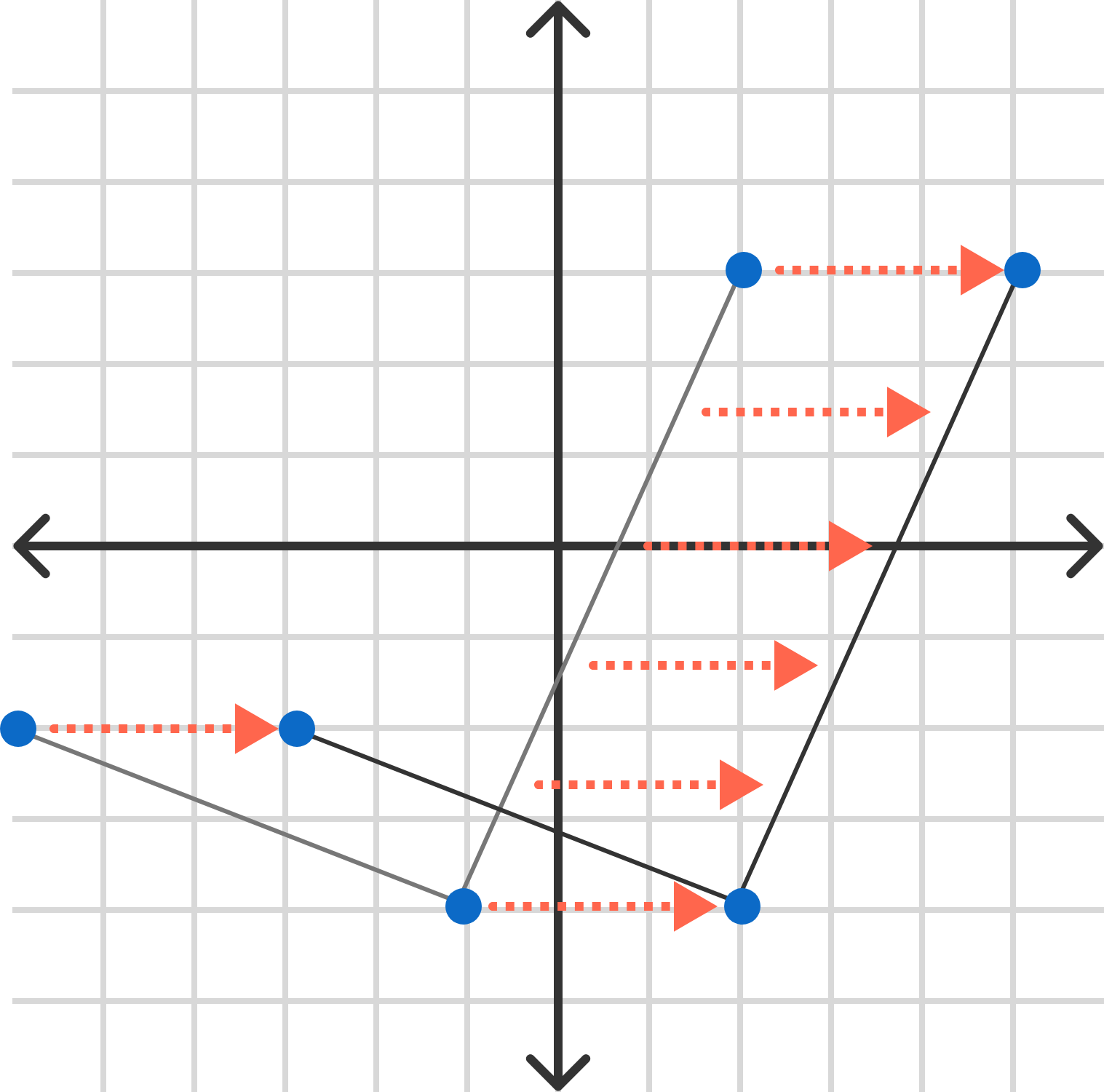If you've gone through the Algebra II course, you've seen (during function transformations) how an operation happens "in reverse" if applied before the main function; for example, $$f(x-3)$$ moves a function to the right by $$3$$ rather than to the left, that is, adding 3 to each $$x$$ coordinate rather than subtracting.

With $\left( Qx \right)^2 + y^2 = 1,$ the effect one might expect is again reversed: multiplying by $$Q$$ divides the $$x$$-coordinates.

In order to "un-reverse" the effect, the multiplication by a constant is usually written as division. That is, we could make a new variable $$a = \frac{1}{Q}$$ and substitute to get $\left( \frac{x}{a} \right)^2 + y^2 = 1$ or $\frac{x^2}{a^2} + y^2 = 1 .$ Now, quite conveniently, the value of $$a$$ directly matches the amount the horizontal is stretched out; $$a = 4$$ will make an ellipse that intersects at $$(4,0)$$ and $$(-4,0),$$ as shown below.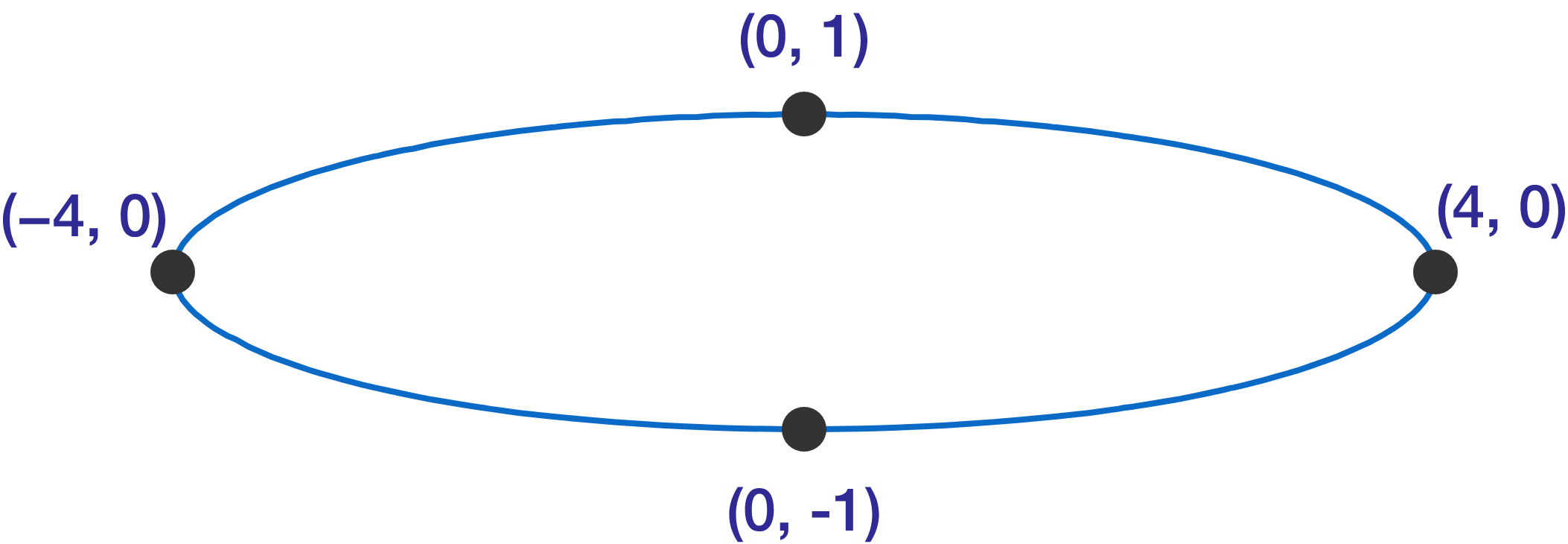## Orbits

### Introduction

# Orbits

Which graph matches that of $\frac{x^2}{16} + \frac{y^2}{4} = 1 ?$ Notice we've now introduced vertical stretching as well; feel free to reason out how it works or just use your intuition.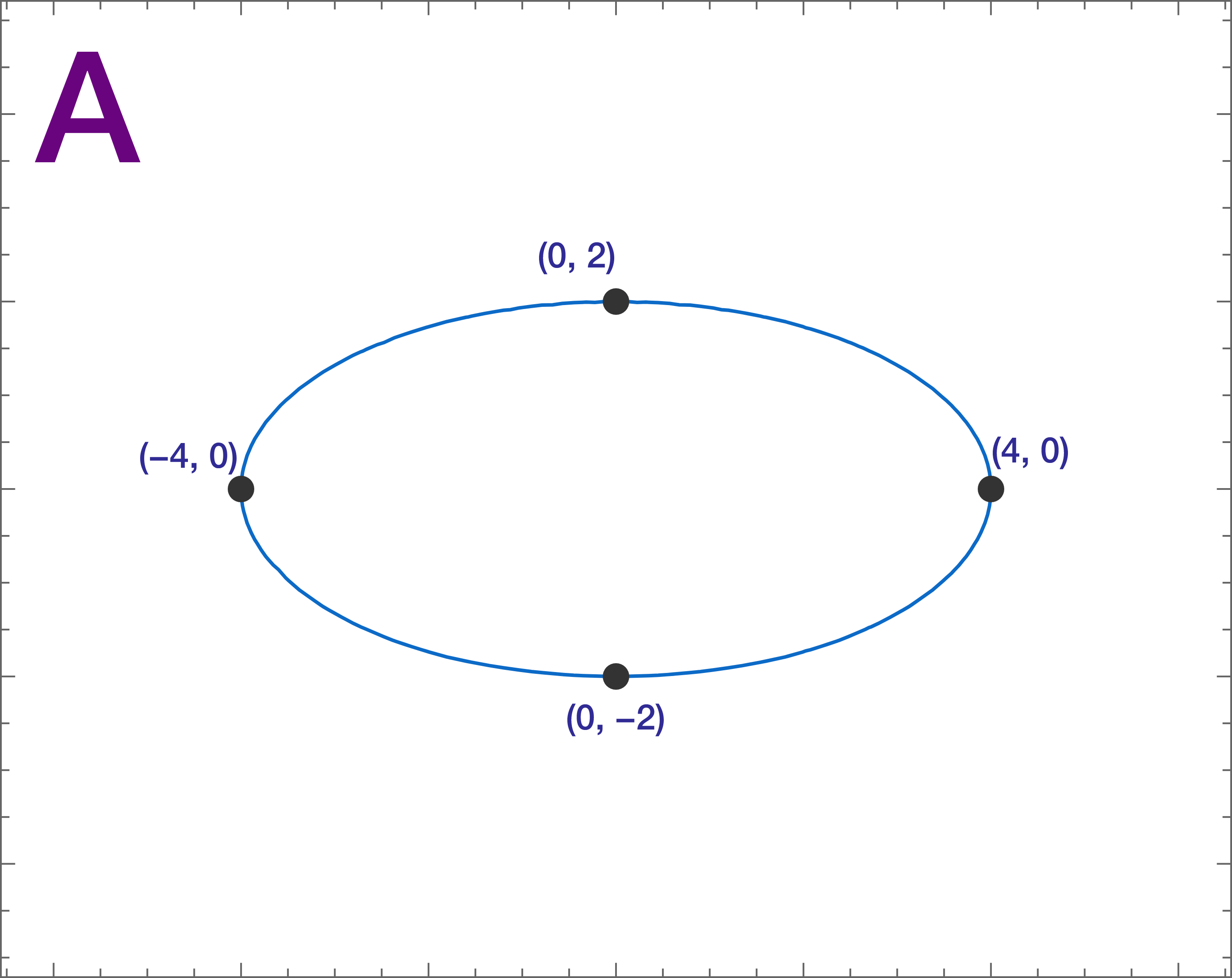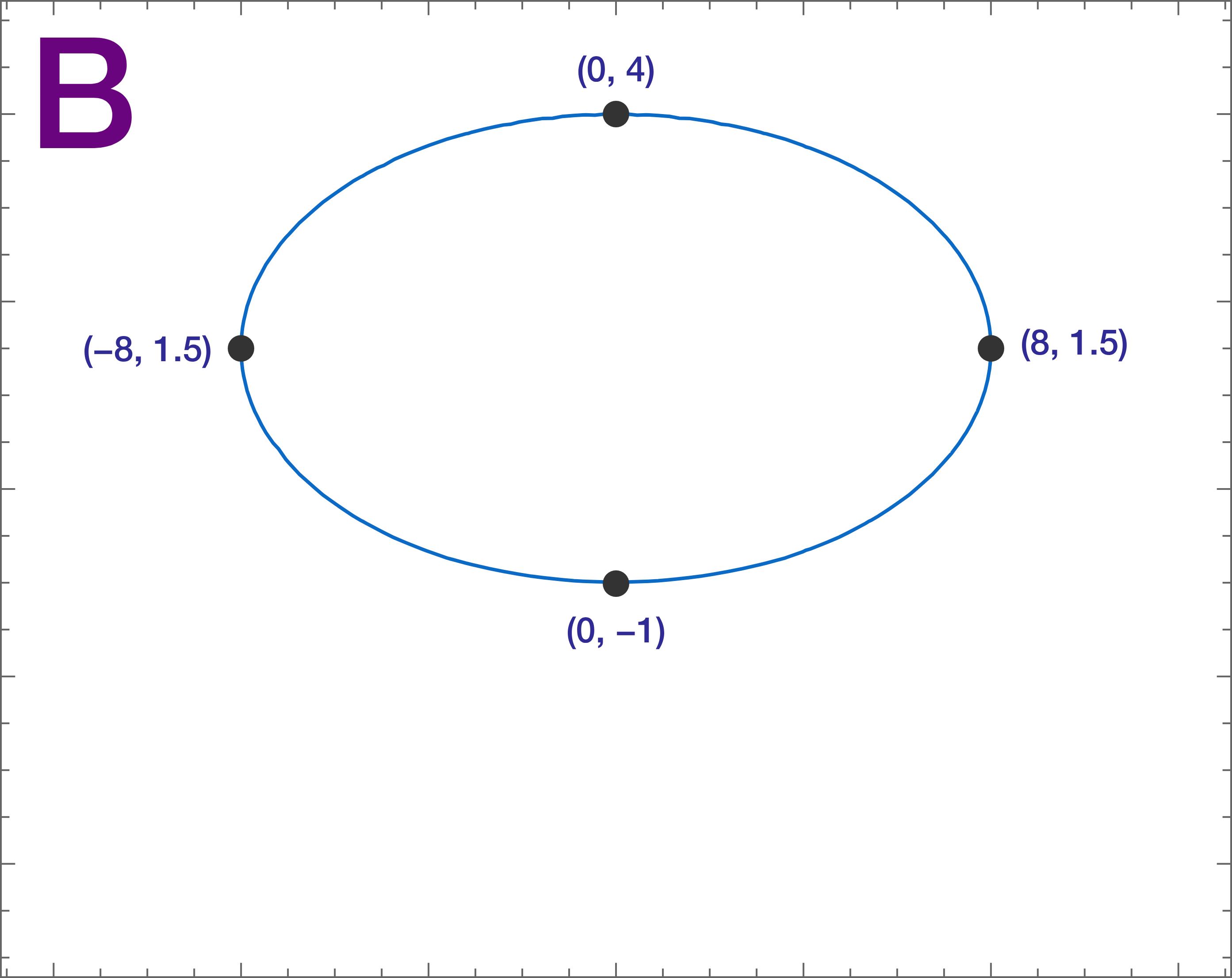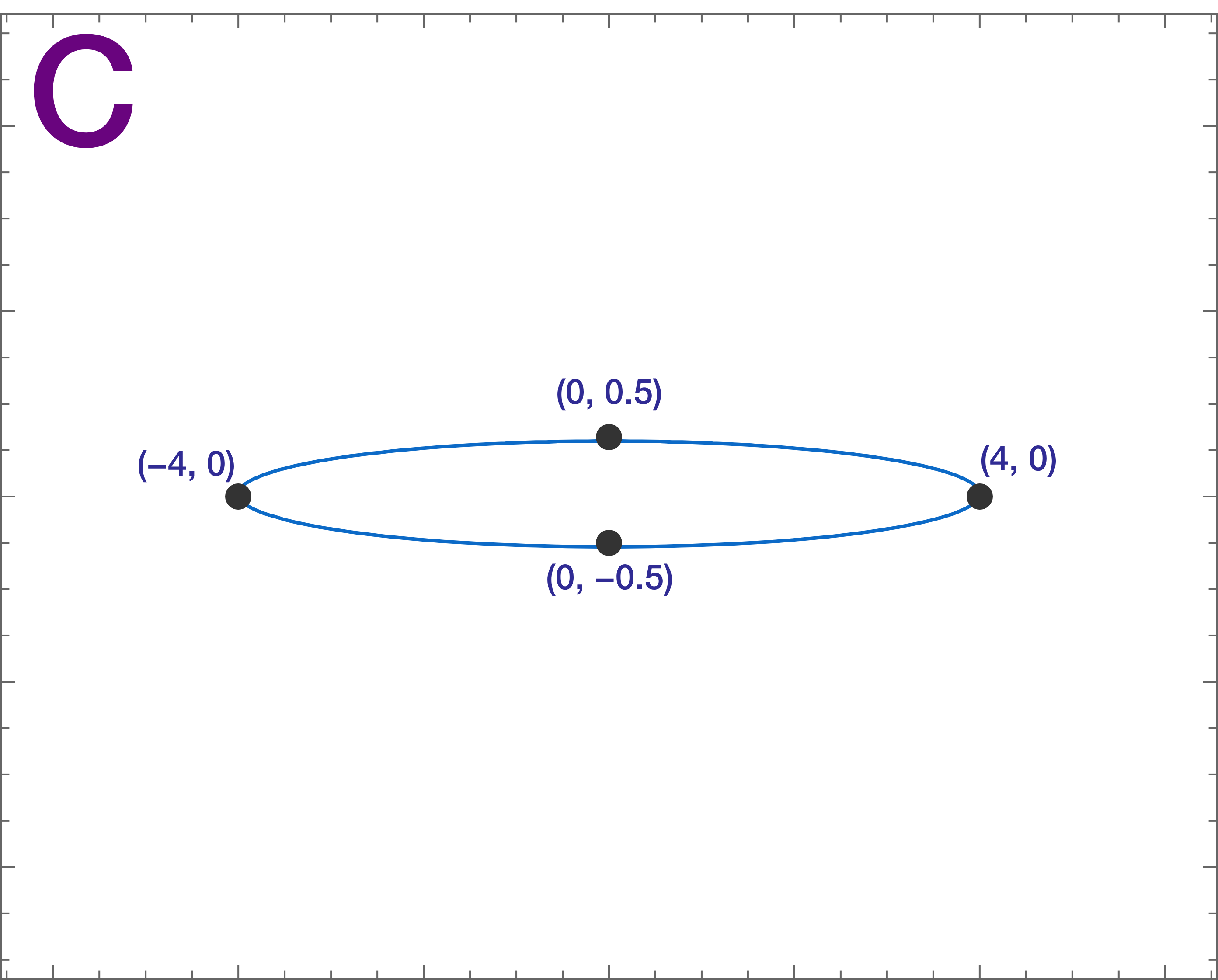## Orbits

### Introduction

# Orbits

Applying what you saw in the previous problem, the general formula for an ellipse can be written as $\frac{x^2}{a^2} + \frac{y^2}{b^2} = 1.$ What happens when $$a = b ?$$

## Orbits

### Introduction

# Orbits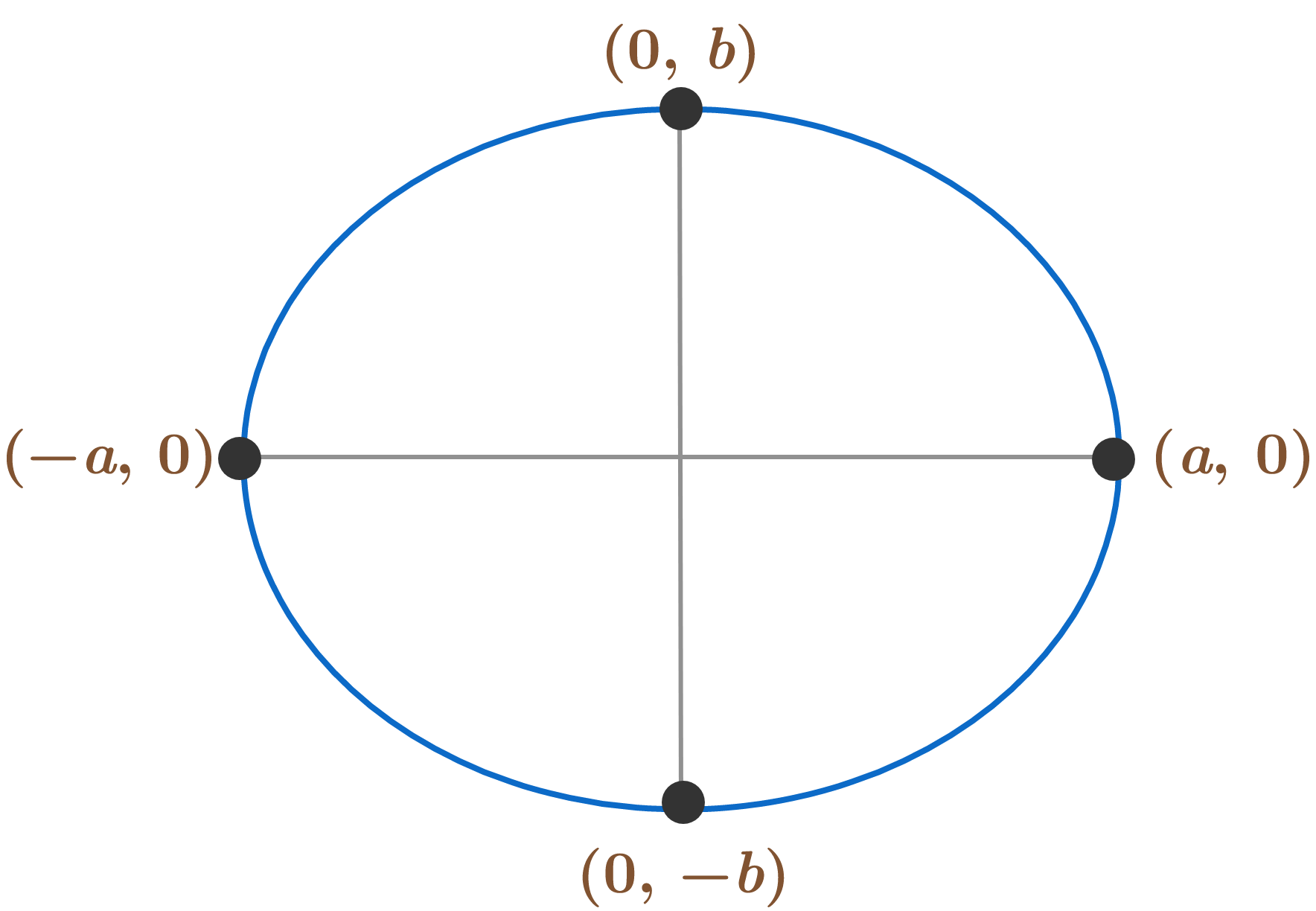$\frac{x^2}{a^2} + \frac{y^2}{b^2} = 1$ Feel free to experiment with the ellipse equation before moving on. Note it is possible, as you'll see in a later question of this quiz, for the ellipse to not be in the center, but for now we'll be sticking with this form.

## Orbits

### Introduction

# Orbits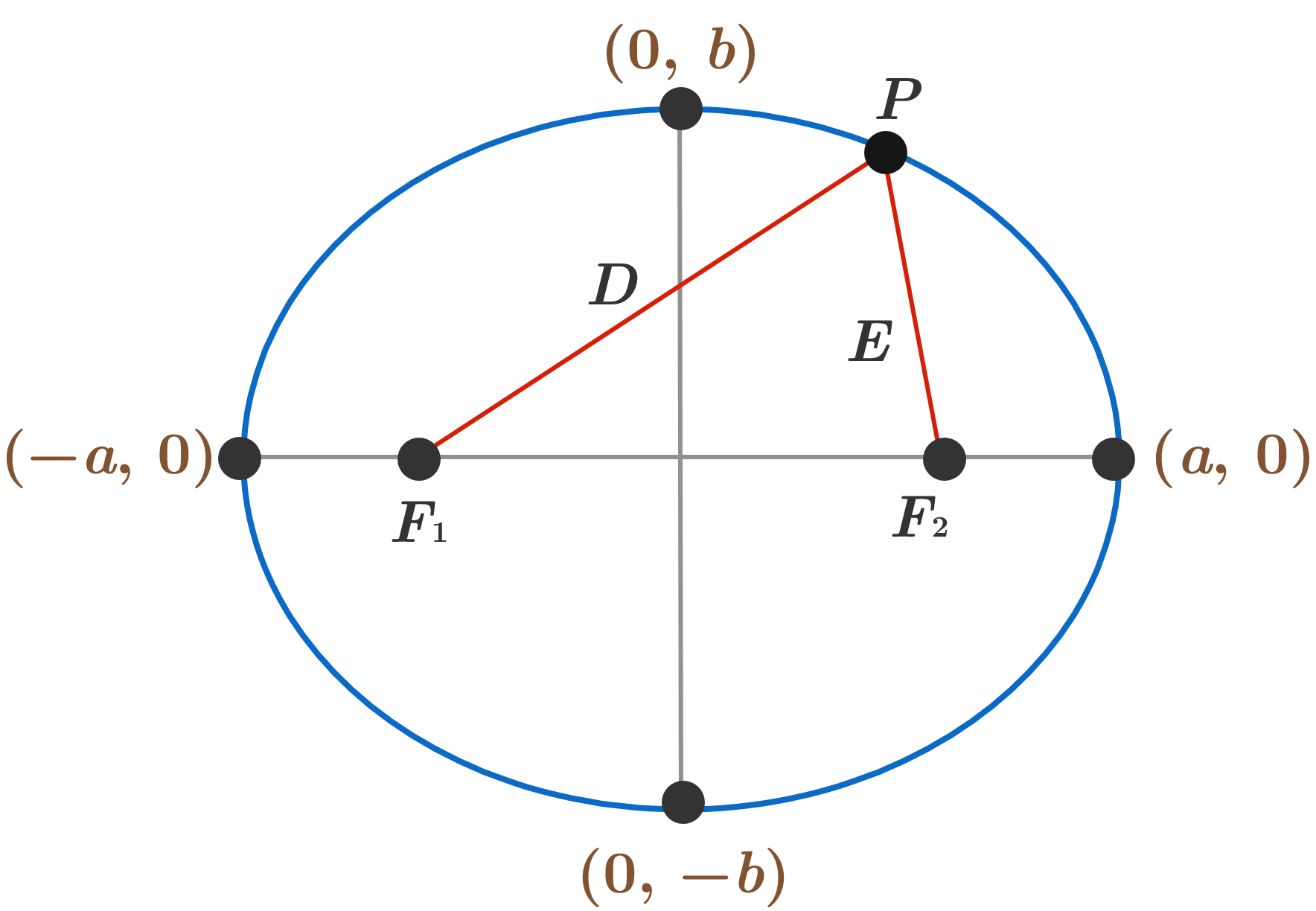Going back to the original definition, the distance $$D + E$$ as marked above will be the same for every point $$P$$ on the ellipse. $$F_1$$ and $$F_2$$ are the foci.

We're going to assume $$a$$ and $$b$$ are defined as in the previous few problems, and also suppose $$a > b .$$ Given this situation, which equation is true?

Note: We are assuming $$a >b$$ to simplify things so our major axis (the longer one) will be on the $$x$$-axis and minor axis will be on the $$y$$-axis. The foci land on the major axis, so the diagram would be turned if $$b > a .$$

## Orbits

### Introduction

# Orbits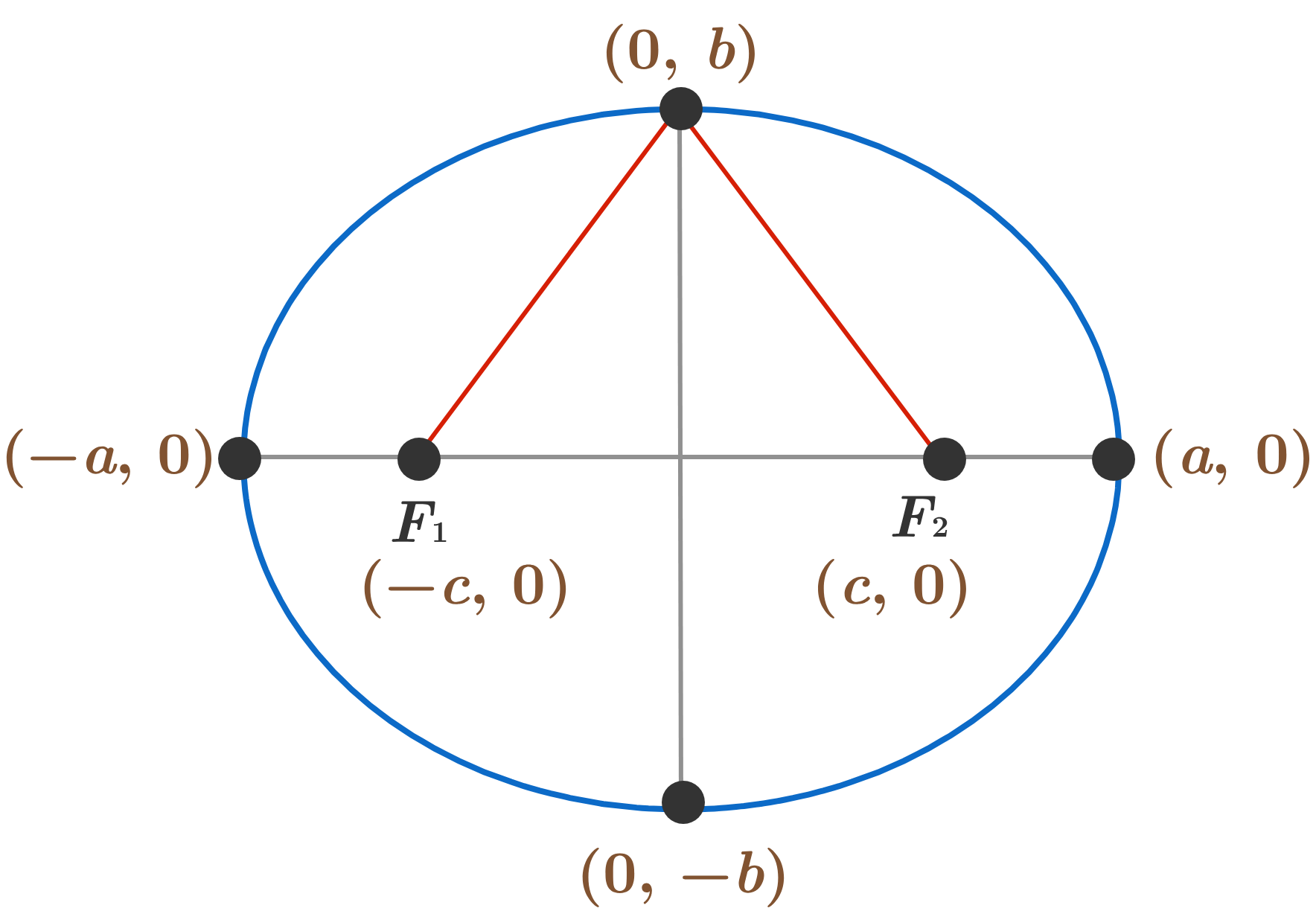Based on the previous statement, we can figure out given $$a$$ and $$b$$ where $$c$$ (as marked on the diagram) is located. What's the correct equation? You can use the diagram shown to puzzle out the answer. Remember we are assuming $$a > b .$$

Note: This solution also includes the full justification that the formula we've derived by stretching a circle does match the definition of an ellipse.

## Orbits

### Introduction

# Orbits

In this visualization, one focal point of an ellipse is fixed at the origin; the sliders move the other focal point at $$(x_2, y_2) ,$$ changing the path of the orbit. (The "play" button will also let you see the planet in motion!)

Assume the ratio between the distance between the foci and the length of the major axis (also known as the eccentricity) is constant. Which of the two points (2,2) and (3,1) will produce the bigger length of ellipse if used as the second focal point of the ellipse?

## Orbits

### Introduction

# Orbits

Exoplanets are planets outside of our solar system. The exoplanet 61 Virginis-d 9 (orbiting the star 61 Virginis in the constellation Virgo) has the conic equation $4x^2 + 5y^2 =1.$ The sun will be at one of the focal points while the planet traces the path of the ellipse. How far away is the planet from its sun when the two are at their closest? (For exoplanets the term is periastron.)

Note: Measurements are in AU (astronomical units), which is defined as the average distance between Earth and the sun.

## Orbits

### Introduction

# Orbits

As you've experienced through this introduction, trigonometry and conic sections appear prolifically in natural, technological, and engineering applications.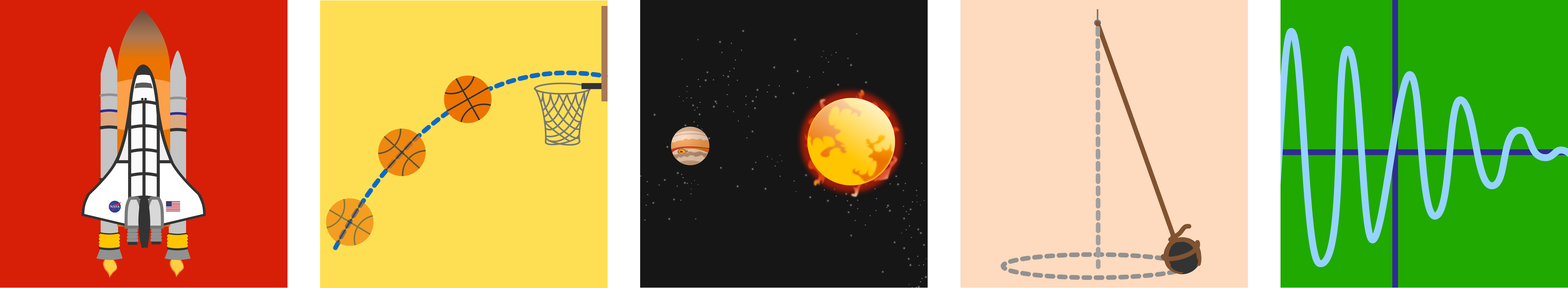Included in this course are

• circles and ellipses, the curves which describe planetary and satellite orbits;
• parabolas, which model projectile motion and the shape of satellite dishes;
• the sine, cosine, and tangent functions which govern the behavior of all harmonic oscillators including springs, pendulums, sound waves, and light waves;
• logarithms and exponentials which can describe growth and decay in both biology and finance.

Start your journey with conic sections!

## Orbits

### Introduction

×